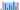# Container with Most Water - Leet Code Solution

September 13, 2019## Problem Statement

Given n non-negative integers a1, a2, …, an , where each represents a point at coordinate (i, ai). n vertical lines are drawn such that the two endpoints of line i is at (i, ai) and (i, 0). Find two lines, which together with x-axis forms a container, such that the container contains the most water.

Note: You may not slant the container and n is at least 2.

## Algorithm-1 (Brute force)

``````public int maxArea(int[] height) {
int max = 0;
int l = height.length;

for (int i=0; i<l; i++) {
for (int j=i+1; j<l; j++) {
int area = (j-i) * Math.min(height[i], height[j]);
if (area > max) {
max = area;
}
}
}

return max;
}``````

## Algorithm-2

Lets think of optimizing this computation. What if we start with max range, and move either left or right. Since, lower height determines the area. And, we can move greedily towards point with high height. And, compare the area.

We can start with left and right pointer, calculate area. Then we can move towards point with higher height. i.e. the side which is having less height, we should move that pointer. Example: If left pointer value is less, move it right. Similarly, if right pointer value is less than right, move it left.

``````public int maxArea(int[] height) {
int l = height.length;
int result = 0;
int i=0;
int j = l-1;

while (i < j) {
int area = (j-i) * Math.min(height[i], height[j]);
if (result < area) {
result = area;
}

if (height[i] < height[j]) {
i++;
}
else j--;
}

return result;
}``````